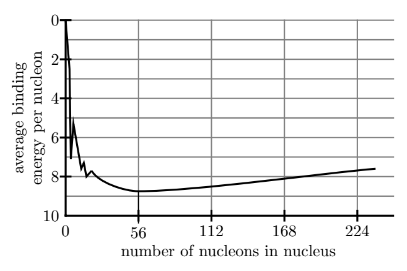# Problem: Observe the graph of the binding energy per nucleon shown to the right. Based on the graph, which of the following statements is true? A. One should expect that fusion type reactions that occur with nuclides with less than 56 nucleons should be exothermic.B. One should expect that fission type reactions that occur with nuclides with less than 56 nucleons should be exothermic.C. One should expect that fusion reactions that occur with nuclides with more than 56 nucleons should be exothermic.D. One should expect that fission reactions that occur with nuclides with more than 56 nucleons should be endothermic.

###### FREE Expert Solution

We're being asked to determine which of the given statements is true.

Let's first define nuclear fission and nuclear fusion.

Nuclear Fission is the splitting of a heavy nucleus to form two or lighter ones.

Nuclear fusion is a nuclear reaction in which two or more atomic nuclei combine to form one or larger atomic nuclei and subatomic particles.

$\overline{){\mathbf{∆}}{\mathbf{E}}{\mathbf{=}}{{\mathbf{E}}}_{{\mathbf{products}}}{\mathbf{-}}{{\mathbf{E}}}_{{\mathbf{reactants}}}}$

Now let's analyze each statement given:

80% (493 ratings)###### Problem Details

Observe the graph of the binding energy per nucleon shown to the right. Based on the graph, which of the following statements is true?

A. One should expect that fusion type reactions that occur with nuclides with less than 56 nucleons should be exothermic.

B. One should expect that fission type reactions that occur with nuclides with less than 56 nucleons should be exothermic.

C. One should expect that fusion reactions that occur with nuclides with more than 56 nucleons should be exothermic.

D. One should expect that fission reactions that occur with nuclides with more than 56 nucleons should be endothermic.